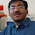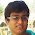### Number of digits in 125^100

Source: Asked to me by Suman Kalyan Bera (M.Tech  Student IIT Delhi) on contact page

Problem:

How many digits does the number 125^100 have?

Very interesting problem and equally interesting solution :)

Update (25 December 2011):
You are not allowed to use your high school notes on values of log_10(2) or log_10(5)
Solution:

1.solution :P
#include< cstdio >
#include< sstream >
#include< cstdlib >
#include< cctype >
#include< cmath >
#include< algorithm >
#include< set >
#include< queue >
#include< stack >
#include< list >
#include< iostream >
#include< fstream >
#include< numeric >
#include< string >
#include< vector >
#include< cstring >
#include< map >
#include< iterator >
#include< bitset >
using namespace std;
#define sf scanf
#define pf printf
#define re return
#define i64 __int64
#define I64 %I64d
#define u64 unsigned __int64
#define U64 "%U64d"
#define ll long long
#define forit(i, m) for (i=(m).begin(); i!=(m).end(); ++i)
#define rab(i,a,b) for(i=a;i<=b;i++)
#define ras(i,a,b) for(i=a;i>=b;i--)
#define rep(i,n) rab(i,0,n-1)
#define fi(n) rep(i,n)
#define fj(n) rep(j,n)
#define fk(n) rep(k,n)
#define max(a,b) a>b? a:b
#define min(a,b) aeps)
#define inf (1<<30)
#define pi 2*acos(0.0)
#define Set(t,v) memset((t), (v), sizeof(t))
#define pb push_back
#define sz(v) (v).size()
#define vt vector
#define mp make_pair
#define all(x) x.begin(),x.end()
#define rev(x) reverse(all(x))
#define im int main()
#define sq(a) (a)*(a)
#define max 1000001
ll gcd(ll a,ll b){while(b){b ^=a ^=b ^=a %=b;}return a;}
im
{
char a[max];
sf("%s",&a);
pf("%d\n",strlen(a));

re 0;
}

2.The number of digits in a number x equal log of x (to the base 10)
In this case 100*log(125)

3.log_10(125^100) = 209.691001
Hence 209 digits

4.taking log(base 10) of 125^100 it gives the number of digit it contains

5.125^100 = 5^300. We know log5 is 1-log2 = 0.6990 (from my JEE prep days). 300*.699 = 209.7. Therefore 210 digits. Yeh bhee koi question hai?!? Or am I missing something?

6.The number of digits is floor(log_10 125^100) + 1 = floor (log_10 5^300) + 1 = floor(300(1-log_10 2)) + 1 which is to enough digits of accuracy, floor (300 - 300*0.3010) + 1 = 210.

But, certainly the fact that I knew log_10 2 is roughly 0.3010 came in handy here.

7.300, I'd say.

8.K=125^100.
No.of Digits=floor(log K)+1
log(K)= 300-100*log8=300-300*log2=209.3
Ans=210.

9.isn't is just solving for n where n satisfies:

10^(n+1)>= 125^100 >= 10^n

n solves to 209
thus 210 digits long (google calculator says its right)

10.Ok. May be I should have been clear. Most people do not know what log_10(2) or log_10(5) is!

You have to find the answer without using your high school notes, by pure logic.

Solution:

125^100 = 1000^100/(2^300)

2^300 = 1024^30

125^100 = 1000^70/(1.024^30)

Claim: 1 < 1.024^30 < 10

So, 10^209 < 125^100 < 10^210

Number of digits = 210 :)

11.Regarding my claim about 1.024^30 < 10

(1+x)^30 = 1 + 30x + 30C2*x*x + 30C3*x*x*x + ...

Each subsequent term is a factor of less than 30C(i+1)*x/30C(i) of the previous term, i.e.
x*(30-i)/(i+1) < 30x for all i

30x = y = 0.72

(1+x)^30 < 1 + y + y*y + ... 31 terms

(1+x)^30 < 1/(1-y) < 10

Hence, done

@khalil, nick, mani, sid, circem, avinash, siva

I guess all of you did the same thing, with some mistakes at some places, but the argument was pretty much the same. Thanks

@Anik,
how is this the solution?

12.another way of proving the claim which doesn't require knowledge of binomial theorem :-
(1.024)^30 < 10
it is sufficient if we prove (1.024)^32 < 10
it would be sufficient if we prove
(1.024)^16 <3
it wud be sufficient if we prove
(1.024)^8<1.7
it wud be sufficient if we prove
(1.024)^4 < 1.3
it wud be sufficient if we prove
(1.05)^2 < 1.3 (by squaring 1.024)
1.1025< 1.3 which is true.